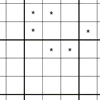# Resources tagged with: Working systematically

Filter by: Content type:
Age range:
Challenge level:

### There are 132 results

Broad Topics > Thinking Mathematically > Working systematically### Product Sudoku

##### Age 11 to 16Challenge Level

The clues for this Sudoku are the product of the numbers in adjacent squares.### LCM Sudoku

##### Age 14 to 16Challenge Level

Here is a Sudoku with a difference! Use information about lowest common multiples to help you solve it.### American Billions

##### Age 11 to 14Challenge Level

Play the divisibility game to create numbers in which the first two digits make a number divisible by 2, the first three digits make a number divisible by 3...### Multiples Sudoku

##### Age 11 to 14Challenge Level

Each clue in this Sudoku is the product of the two numbers in adjacent cells.### A First Product Sudoku

##### Age 7 to 14Challenge Level

Given the products of adjacent cells, can you complete this Sudoku?### Ben's Game

##### Age 11 to 16Challenge Level

Ben, Jack and Emma passed counters to each other and ended with the same number of counters. How many did they start with?##### Age 11 to 14Challenge Level

If you take a three by three square on a 1-10 addition square and multiply the diagonally opposite numbers together, what is the difference between these products. Why?### How Old Are the Children?

##### Age 11 to 14Challenge Level

A student in a maths class was trying to get some information from her teacher. She was given some clues and then the teacher ended by saying, "Well, how old are they?"### Cayley

##### Age 11 to 14Challenge Level

The letters in the following addition sum represent the digits 1 ... 9. If A=3 and D=2, what number is represented by "CAYLEY"?### Two and Two

##### Age 11 to 16Challenge Level

How many solutions can you find to this sum? Each of the different letters stands for a different number.##### Age 11 to 14Challenge Level

By selecting digits for an addition grid, what targets can you make?### Gabriel's Problem

##### Age 11 to 14Challenge Level

Gabriel multiplied together some numbers and then erased them. Can you figure out where each number was?##### Age 11 to 14Challenge Level

Replace the letters with numbers to make the addition work out correctly. R E A D + T H I S = P A G E### Star Product Sudoku

##### Age 11 to 16Challenge Level

The puzzle can be solved by finding the values of the unknown digits (all indicated by asterisks) in the squares of the $9\times9$ grid.### Football Sum

##### Age 11 to 14Challenge Level

Find the values of the nine letters in the sum: FOOT + BALL = GAME### Diagonal Product Sudoku

##### Age 11 to 16Challenge Level

Given the products of diagonally opposite cells - can you complete this Sudoku?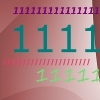### Ones Only

##### Age 11 to 14Challenge Level

Find the smallest whole number which, when mutiplied by 7, gives a product consisting entirely of ones.### LCM Sudoku II

##### Age 11 to 18Challenge Level

You are given the Lowest Common Multiples of sets of digits. Find the digits and then solve the Sudoku.### Peaches Today, Peaches Tomorrow...

##### Age 11 to 14Challenge Level

A monkey with peaches, keeps a fraction of them each day, gives the rest away, and then eats one. How long can his peaches last?### Factor Lines

##### Age 7 to 14Challenge Level

Arrange the four number cards on the grid, according to the rules, to make a diagonal, vertical or horizontal line.##### Age 11 to 16Challenge Level

The items in the shopping basket add and multiply to give the same amount. What could their prices be?### Charitable Pennies

##### Age 7 to 14Challenge Level

Investigate the different ways that fifteen schools could have given money in a charity fundraiser.### Special Numbers

##### Age 11 to 14Challenge Level

My two digit number is special because adding the sum of its digits to the product of its digits gives me my original number. What could my number be?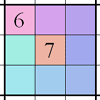### Integrated Product Sudoku

##### Age 11 to 16Challenge Level

This Sudoku puzzle can be solved with the help of small clue-numbers on the border lines between pairs of neighbouring squares of the grid.### Where Can We Visit?

##### Age 11 to 14Challenge Level

Charlie and Abi put a counter on 42. They wondered if they could visit all the other numbers on their 1-100 board, moving the counter using just these two operations: x2 and -5. What do you think?### Sticky Numbers

##### Age 11 to 14Challenge Level

Can you arrange the numbers 1 to 17 in a row so that each adjacent pair adds up to a square number?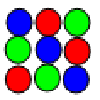### Latin Squares

##### Age 11 to 18

A Latin square of order n is an array of n symbols in which each symbol occurs exactly once in each row and exactly once in each column.##### Age 7 to 14Challenge Level

I added together some of my neighbours' house numbers. Can you explain the patterns I noticed?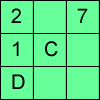### Alphabetti Sudoku

##### Age 11 to 16Challenge Level

This Sudoku requires you to do some working backwards before working forwards.### Consecutive Negative Numbers

##### Age 11 to 14Challenge Level

Do you notice anything about the solutions when you add and/or subtract consecutive negative numbers?### Cuboids

##### Age 11 to 14Challenge Level

Can you find a cuboid that has a surface area of exactly 100 square units. Is there more than one? Can you find them all?### Weights

##### Age 11 to 14Challenge Level

Different combinations of the weights available allow you to make different totals. Which totals can you make?### Making a Difference

##### Age 11 to 14Challenge Level

How many different differences can you make?### Consecutive Numbers

##### Age 7 to 14Challenge Level

An investigation involving adding and subtracting sets of consecutive numbers. Lots to find out, lots to explore.### An Introduction to Magic Squares

##### Age 7 to 16### Cinema Problem

##### Age 11 to 14Challenge Level

A cinema has 100 seats. Show how it is possible to sell exactly 100 tickets and take exactly Â£100 if the prices are Â£10 for adults, 50p for pensioners and 10p for children.### Summing Consecutive Numbers

##### Age 11 to 14Challenge Level

15 = 7 + 8 and 10 = 1 + 2 + 3 + 4. Can you say which numbers can be expressed as the sum of two or more consecutive integers?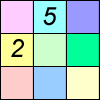### Difference Sudoku

##### Age 14 to 16Challenge Level

Use the differences to find the solution to this Sudoku.### Pole Star Sudoku 2

##### Age 11 to 16Challenge Level

This Sudoku, based on differences. Using the one clue number can you find the solution?### Maths Trails

##### Age 7 to 14

The NRICH team are always looking for new ways to engage teachers and pupils in problem solving. Here we explain the thinking behind maths trails.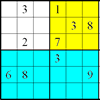### Colour Islands Sudoku

##### Age 11 to 14Challenge Level

An extra constraint means this Sudoku requires you to think in diagonals as well as horizontal and vertical lines and boxes of nine.### Number Daisy

##### Age 11 to 14Challenge Level

Can you find six numbers to go in the Daisy from which you can make all the numbers from 1 to a number bigger than 25?### The Great Weights Puzzle

##### Age 14 to 16Challenge Level

You have twelve weights, one of which is different from the rest. Using just 3 weighings, can you identify which weight is the odd one out, and whether it is heavier or lighter than the rest?### Window Frames

##### Age 5 to 14Challenge Level

This task encourages you to investigate the number of edging pieces and panes in different sized windows.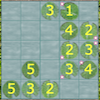### Latin Lilies

##### Age 7 to 14Challenge Level

In this game you are challenged to gain more columns of lily pads than your opponent.### Squares in Rectangles

##### Age 11 to 14Challenge Level

A 2 by 3 rectangle contains 8 squares and a 3 by 4 rectangle contains 20 squares. What size rectangle(s) contain(s) exactly 100 squares? Can you find them all?### Pair Sums

##### Age 11 to 14Challenge Level

Five numbers added together in pairs produce: 0, 2, 4, 4, 6, 8, 9, 11, 13, 15 What are the five numbers?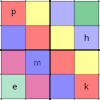### Simultaneous Equations Sudoku

##### Age 11 to 16Challenge Level

Solve the equations to identify the clue numbers in this Sudoku problem.### 9 Weights

##### Age 11 to 14Challenge Level

You have been given nine weights, one of which is slightly heavier than the rest. Can you work out which weight is heavier in just two weighings of the balance?